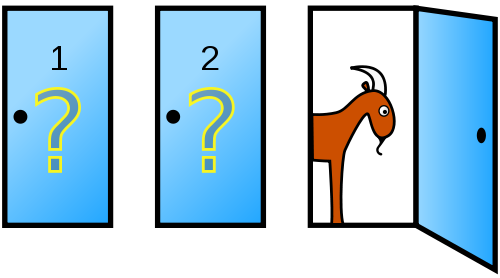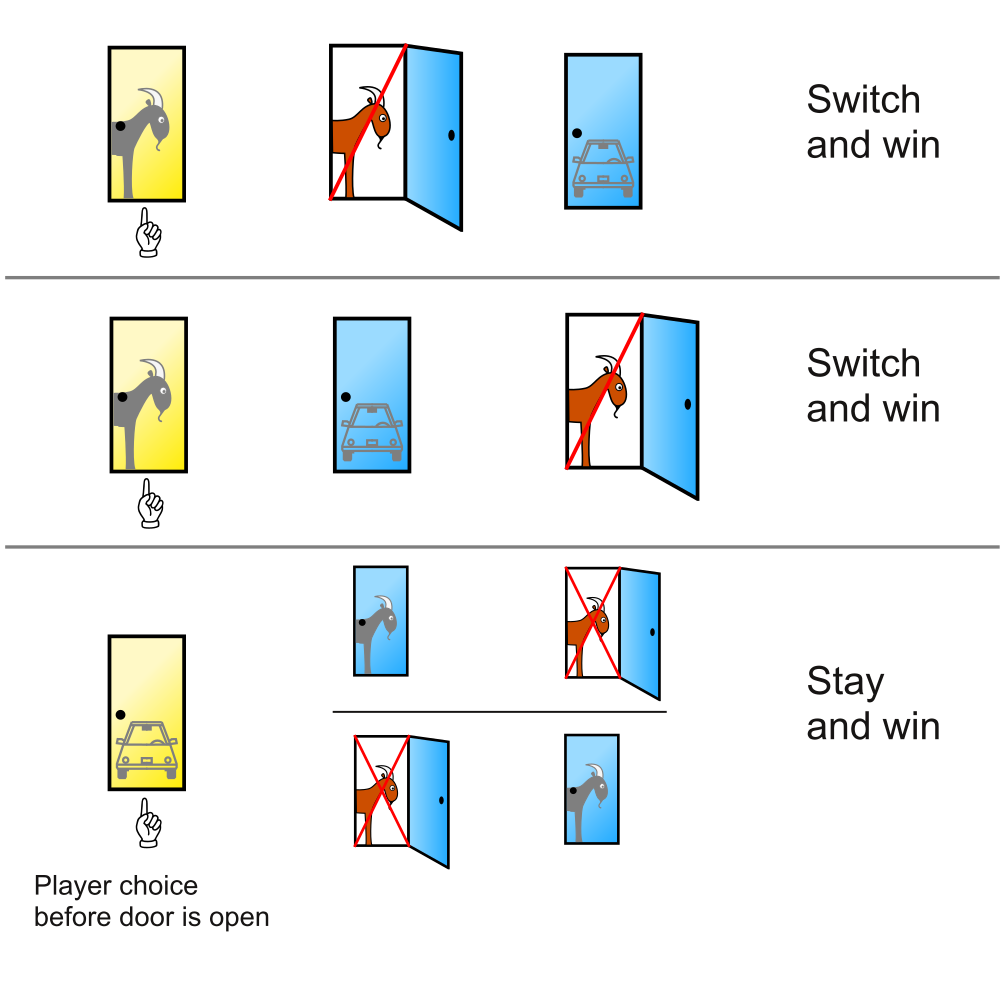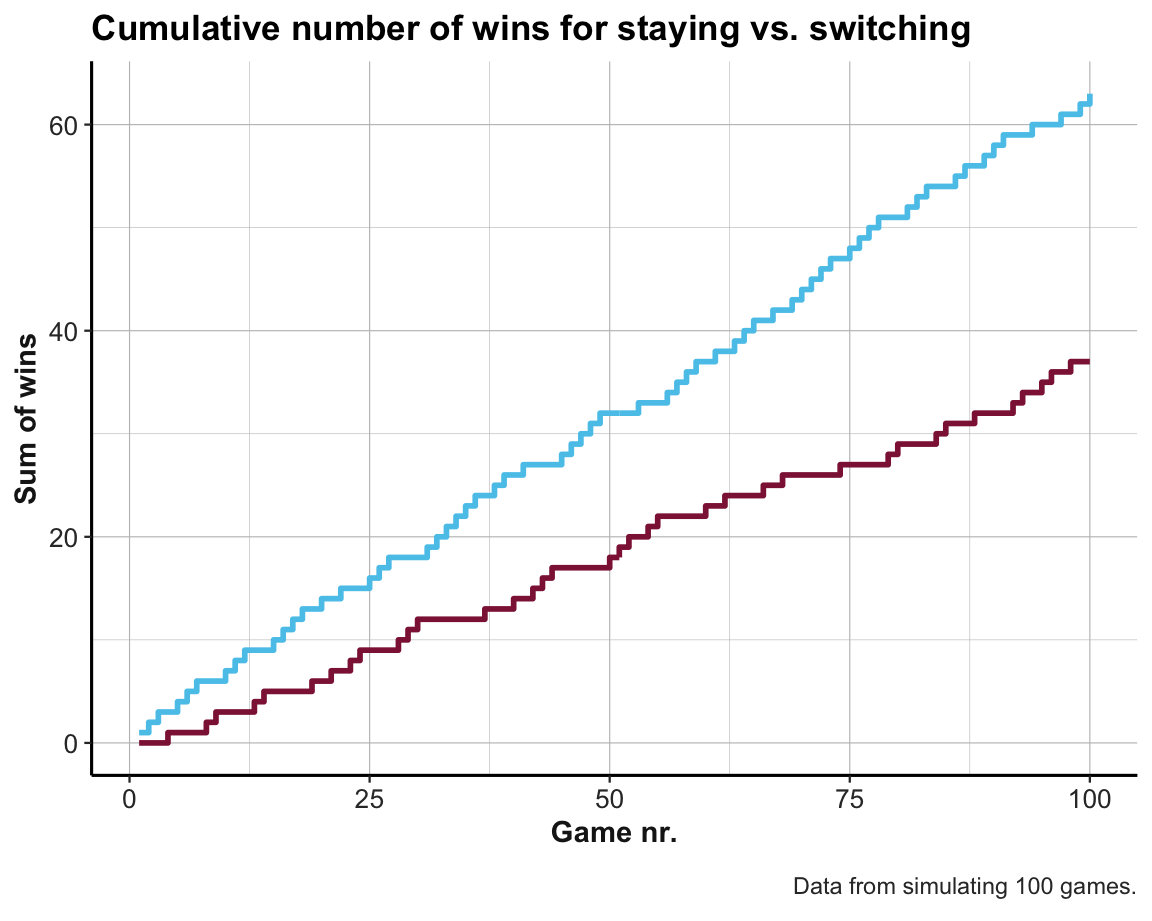## 16.3 Sampling cases

Goal: Taking probabilities more seriously by actually using the probabilities provided in a process of random sampling. This adds complexity (e.g., different runs yield different outcomes) but may also increase the realism our our simulation (as it’s unlikely to obtain perfectly stable results in reality).

Rather than calculate the expected values, we use the probabilities stated in the problem to sample from the appropriate population.

Two important aspects of managing randomness:

• Use set.seed() for reproducible randomness.
• Assess the robustness of results based on repetitions.

### 16.3.1 The Monty Hall problem

A notorious brain teaser problem is based on the US-American TV show Let’s make a deal (see Selvin, 1975). The show involves that a lucky player faces a choice between three doors. It is known that one door hides the grand prize of a car (i.e., win), whereas the other two hide goats (i.e., losses). After choosing a closed door (e.g., Door 1), the show’s host (named Monty Hall) intervenes by opening one of the unchosen doors to reveal a goat. He then offers the player the option to switch to the other closed door. Should the player switch to the other door? The player’s situation is illustrated by Figure 16.3.Figure 16.3: The Monty Hall problem from the player’s perspective. (Source: Illustration from Wikipedia: Monty Hall problem.)

#### The problem

The following version of the dilemma was famously discussed by :

Suppose you’re on a game show, and you’re given the choice of three doors:
Behind one door is a car; behind the others, goats.
You pick a door, say No. 1, and the host, who knows what’s behind the doors,
opens another door, say No. 3, which has a goat.
He then says to you, “Do you want to pick door No. 2?”

Note that the correct answer to the question asked is either “yes” or “no.” Answering “yes” implies that switching doors is generally better than staying with the initial choice. By contrast, answering “no” implies that staying is generally at least not worse than switching doors.

### 16.3.2 Analysis

This problem and its variants have been widely debated — and even revealing its solution is often met with disbelief and likely to spark controversy.

Most people intuitively assume that — given Monty Hall’s intervention — the player faces a 50:50 chance of winning and it therefore makes no difference whether she sticks with her initial choice or switches to the other door. (Note that this does not yet justify why the majority of people prefer to stick with their initial door, but we could postulate a variety of so called “biases” or psychological mechanisms for this preference.)

However, the correct answer to the question is “yes”: The player is more likely to win the car if she always switches to the alternative door. There are many possible ways to explain this:

• Perhaps the simplest explanation asks: What is the probability of winning the car with the player’s initial choice (i.e., without any other interactions)? Most people would agree that $$p(win\ with\ d_i) = \frac{1}{3}$$ for any arbitrary door $$d_i$$. Accepting that Monty Hall’s actions cannot possibly change this (as he cannot transfer the car to a different location), we should conclude that the winning chance for sticking with the initial choice also is $$p(stay) = \frac{1}{3}$$ and $$p(switch) = 1 - p(stay) = \frac{2}{3}$$. (The subtle reason for the benefit for switching is that Monty Hall must take the car’s location into account to reliably open a door that reveals a goat. Thus, Monty Hall curates the choices in a way that adds information.)

• A model-based approach could visualize the three possible options for the car’s location (which are known to be equiprobable, given the car’s random allocation). By explicating the consequences of an initial choice (e.g., of Door 1), we see that always sticking with the initial choice has a theoretical chance of winning in $$p(stay) = \frac{1}{3}$$ of cases. By contrast, always switching to the alternative door provides a higher change of winning of $$p(switch) = \frac{2}{3}$$ (see Figure 16.4).Figure 16.4: An explanation for the superiority of switching in the Monty Hall problem. (Source: Illustration from Wikipedia: Monty Hall problem.)

Many people find the correct solution so counterintuitive that they are unwilling to accept these explanations. If someone refuses to accept the theoretical arguments, an alternative way of convicing them is by simulating a large number of games and then compare the success of either staying or switching doors.14

### 16.3.3 Representing the environment

Our task is to use simulations to decide and justify whether the the contestant should stay or switch. More precisely, is the probability of winning the game by switching larger than by staying with the initial door? What are the probabilities for winning the car in both cases?

To solve this task, we create a simulation with the following features: 3 doors, random location of the car, Monty knows the car’s location and always opens a door that reveals a goat. (Exercise 16.5.3 will extend this solution to some variants of the problem.)

#### Preparations

The most important element for any simulation is to create a valid model of the game scenario.

We will first create a data structure that can represent the setups for N games:

# Generate a random setup:
setup <- sample(x = c("car", "goat", "goat"), size = 3, replace = FALSE)
setup
#>  "car"  "goat" "goat"

# Create N games (with a column for each door):
N <- 100
games <- NA

# Prepare data structure:
games <- tibble::tibble(d1 = rep("NA", N),
d2 = rep("NA", N),
d3 = rep("NA", N))

#### Sampling setups

We now create N random setups and store them in our prepared table of games:

# Fill table with random games:
set.seed(2468)  # for reproducible randomness

for (i in 1:N){

setup <- sample(x = c("car", "goat", "goat"), size = 3, replace = FALSE)

games$d1[i] <- setup games$d2[i] <- setup
games$d3[i] <- setup } head(games) #> # A tibble: 6 x 3 #> d1 d2 d3 #> <chr> <chr> <chr> #> 1 goat car goat #> 2 goat goat car #> 3 car goat goat #> 4 goat goat car #> 5 car goat goat #> 6 goat goat car Note on set.seed(2468): ensure reproducible randomness. Any value is fine, in principle, but avoid always using the same values. Importantly, using unconstrained randomness can often be a virtue! ### 16.3.4 Abstract solution Our first simulation of the problem is rather abstract insofar as it ignores all details of Monty’s actions. When realizing that the game show host (named Monty) can always open a door with a goat (since there are two of them), we can simulate the outcome of the game without taking his actions into account. We first create an auxiliary function that allows us to determine whether a game has been won. This depends on the contents of the three doors (i.e., the car’s location) and the player’s final choice (i.e., of Door 1, 2, or 3). A game is won whenever the chosen door contains the car: # Given the specific setup of a game, would the chosen door win the car? win_car <- function(d1, d2, d3, choice){ out <- FALSE setup <- c(d1, d2, d3) if (setup[choice] == "car"){ out <- TRUE } return(out) } # Check: win_car("car", "goat", "goat", 1) #>  TRUE win_car("car", "goat", "goat", 2) #>  FALSE win_car("car", "car", "goat", 2) #>  TRUE win_car("car", "car", "goat", 3) #>  FALSE Simulate the outcomes of N games in three steps: 1. Generate N initial door choices: As the player’s initial choices are independent of the game’s setup, we can either always pick the same door (e.g., Door 1) or select a random door (i.e., 1, 2, or 3) in each game. As picking a random door in each game appears more plausible, we use sample() to draw N initial choices and add those as a new variable to games: # 1. Generate and add N initial door choices: sim_1 <- games %>% mutate(init_door = sample(x = 1:3, size = N, replace = TRUE)) Several details of this step are noteworthy: • Our mutate() function to compute init_door (in Step 1.) contained a second call to sample(), but always picking Door 1 should make no difference for the result, as long as each row of games really was created randomly above.15 • In this call to sample(), we specified replace = TRUE to ensure that repeatedly drawing the same door is possible and that sampling $$N>3$$ times from x = 1:3 is possible. • As we set set.seed(2468) above, the first call this instance of sample() will always yield the same sequence of results. However, as we did not fix a new value of set.seed() here, repeating this step multiple times would create different values every time. 1. Determine all wins by staying: Given a specific setup of doors and the player’s initial choice, we can determine whether staying with the initial choice would win the car: # 2. Determine wins by staying: sim_1 <- sim_1 %>% mutate(win_stay = purrr::pmap_lgl(list(d1, d2, d3, init_door), win_car)) Note: An example for different map() functions of the purrr package: # Functions: square <- function(x){ x^2 } expone <- function(x, y){ x^y } # Data: tb <- tibble(n_1 = sample(1:9, 100, replace = TRUE), n_2 = sample(1:3, 100, replace = TRUE)) # map functions to every row of tb: tb %>% mutate(sqr = purrr::map_dbl(.x = tb$n_1, .f = square),  # 1 argument
exp = purrr::map2_dbl(n_1, n_2, expone),         # 2 arguments
sum = purrr::pmap_dbl(list(n_1, n_2, sqr), sum)  # 3+ arguments
)
#> # A tibble: 100 x 5
#>      n_1   n_2   sqr   exp   sum
#>    <int> <int> <dbl> <dbl> <dbl>
#>  1     4     3    16    64    23
#>  2     9     1    81     9    91
#>  3     4     3    16    64    23
#>  4     1     3     1     1     5
#>  5     9     2    81    81    92
#>  6     3     2     9     9    14
#>  7     1     2     1     1     4
#>  8     9     3    81   729    93
#>  9     1     1     1     1     3
#> 10     2     1     4     2     7
#> # … with 90 more rows
1. Determine all wins by switching:

The third and final step may require some explanation: Assuming that Monty knows the car’s location, but always opens a door with a goat, we can conclude that switching doors wins the game in exactly those cases in which the player’s initial choice did not win the game. A simpler way of expressing this is: Switching doors wins the game whenever the initial choice does not succeed.

# 3. Determine wins by switching:
sim_1 <- sim_1 %>%
mutate(win_switch = !win_stay)

Note that we could combine the three steps above in a single mutate() command:

sim_1 <- games %>%
mutate(init_door = sample(1:3, N, replace = TRUE),
win_stay = purrr::pmap_lgl(list(d1, d2, d3, init_door), win_car),
win_switch = !win_stay)
1. Evaluate results:

At this point sim_1 contains all the information that we need. For instance, to compare the result of consistently staying or switching, we simply inspect the final two variables:

head(sim_1)
#> # A tibble: 6 x 6
#>   d1    d2    d3    init_door win_stay win_switch
#>   <chr> <chr> <chr>     <int> <lgl>    <lgl>
#> 1 goat  goat  car           1 FALSE    TRUE
#> 2 car   goat  goat          3 FALSE    TRUE
#> 3 goat  goat  car           2 FALSE    TRUE
#> 4 car   goat  goat          3 FALSE    TRUE
#> 5 goat  goat  car           1 FALSE    TRUE
#> 6 goat  car   goat          2 TRUE     FALSE

# Results for staying vs. switching:
mean(sim_1$win_stay) #>  0.33 mean(sim_1$win_switch)
#>  0.67

As our theoretical analysis has shown, always switching turns out to be about twice as good as always sticking to the initial choice.

### 16.3.5 Detailed solution

A more concrete simulation would also incorporate the details of Monty’s actions:

Note that we can select game setups and determine the locations of the car and goats as follows:

setup <- games[1, ]  # select a specific setup (row in games)
setup

# Determining locations of interest:
which(setup == "car")  # car's location/index
which(setup == "goat") # goat locations

To flesh out the details of a particular game, we first need to create two additional auxiliary functions:

1. Simulate Monty’s actions (i.e., which door is being opened, based on the setup and the player’s initial choice):
# 1. Monty acts as a function of current setup and player_choice:
host_act <- function(d1, d2, d3, player_choice){

door_open <- NA
setup <- c(d1, d2, d3)
ix_goats <- which(setup == "goat")  # indices of goats

# Distinguish 2 cases:
if (setup[player_choice] == "car"){ # player's initial choice would win the car:

door_open <- sample(ix_goats, 1)  # show a random goat (without preference)

} else { # player's initial choice is a goat:

door_open <- ix_goats[ix_goats != player_choice]  # show the other/unchosen goat

}

return(door_open)

}

# Check:
host_act("car", "goat", "goat", 2)  # Monty must open d3
#>  3
host_act("car", "goat", "goat", 3)  # Monty must open d2
#>  2
host_act("car", "goat", "goat", 1)  # Monty can open d2 or d3
#>  2
host_act("car", "goat", "goat", 1)  # Monty can open d2 or d3
#>  2

Note that we enter the contents of the three doors as three distinct arguments (i.e., d1, d2, and d3), rather than as one argument that uses the vector setup. The reason for this is that we later want to use entire rows of games as inputs to the map() family of functions of the purrr package.

1. Identify the door to switch to (based on an initial choice and Monty’s actions):
# 2. To which door would the player switch (based on initial choice and Monty's action):
switch_door <- function(door_init, door_open){

door_switch <- NA
doors <- 1:3

door_switch <- doors[-c(door_init, door_open)]

return(door_switch)

}

# Check:
switch_door(1, 2)
#>  3
switch_door(1, 3)
#>  2
switch_door(2, 1)
#>  3
switch_door(2, 3)
#>  1
switch_door(3, 1)
#>  2
switch_door(3, 2)
#>  1
1. Simulate N games:

Equipped with these functions, we can now generate all details of N games as a single dplyr pipe:

sim_2 <- games %>%
mutate(door_init = sample(1:3, N, replace = TRUE),  # sample initial choices
# door_init = rep(1, N),  # (always pick Door 1 as initial choice)
# door_init = sim_1$init_door, # (use the same choices as above) door_host = purrr::pmap_int(list(d1, d2, d3, door_init), host_act), door_switch = purrr::pmap_int(list(door_init, door_host), switch_door), win_stay = purrr::pmap_lgl(list(d1, d2, d3, door_init), win_car), win_switch = purrr::pmap_lgl(list(d1, d2, d3, door_switch), win_car) ) head(sim_2) #> # A tibble: 6 x 8 #> d1 d2 d3 door_init door_host door_switch win_stay win_switch #> <chr> <chr> <chr> <int> <int> <int> <lgl> <lgl> #> 1 goat car goat 3 1 2 FALSE TRUE #> 2 goat goat car 2 1 3 FALSE TRUE #> 3 car goat goat 2 3 1 FALSE TRUE #> 4 goat goat car 3 2 1 TRUE FALSE #> 5 car goat goat 3 2 1 FALSE TRUE #> 6 goat goat car 2 1 3 FALSE TRUE 1. Evaluate results: # Results for staying vs. switching: mean(sim_2$win_stay)
#>  0.37
mean(sim_2\$win_switch)
#>  0.63

As expected, always switching is still about twice as good as always sticking to the initial choice.

### 16.3.6 Visualizing simulation results

Whenever running a simulation, it is a good idea to visualize its results. To visualize the number of cumulative wins for consistently using either strategy, we first add some auxiliary variables:

# Add game nr. and cumulative sums to sim:
sim_2 <- sim_2 %>%
mutate(nr = 1:N,
cum_win_stay = cumsum(win_stay),
cum_win_switch = cumsum(win_switch))
dim(sim_2)
#>  100  11

Plot the number of wins per strategy (as a step function):

ggplot(sim_2) +
geom_step(aes(x = nr, y = cum_win_switch), color = Seeblau, size = 1) +
geom_step(aes(x = nr, y = cum_win_stay), color = Bordeaux, size = 1) +
labs(title = "Cumulative number of wins for staying vs. switching",
x = "Game nr.", y = "Sum of wins",
caption = paste0("Data from simulating ", N, " games.")) +
theme_ds4psy()Note that the graph shows that both strategies are indistinguishable at first, but increasingly separate as we play more games. Also, there can be quite long stretches of games for which either strategy fails to win.

Also, the results of our abstract and detailed simulations differ although we used the same setup of games for both. This is because we used sample() to determine the player’s initial choice door_init twice. If we wanted to obtain the same results in both simulations, we could sample the player’s initial choices only once for both simulations or use set.seed() to reproduce the same random sequence twice. However, the variation in results is actually informative. Increasing the number of games N will allow us to approximate the theoretically expected values (of $$p(stay) = \frac{1}{3}$$ and $$p(switch) = \frac{2}{3}$$).

#### Practice

• Explain in your own words why the results for our abstract and detailed solutions slightly differ from each other.

• We decided to let the player choose a random initial door in each game. Confirm that simulating the case in which the player always picks Door 1 would yield the same (qualitative) result.

• Adjust the abstract and detailed simulations so that both allow for random elements (e.g., random game setups and random picks of the player’s initial door), but nevertheless yield exactly the same result.

### References

Savant, M. vos. (1990). Ask Marilyn. Parade Magazine, (September 9), p. 15.
Selvin, S. (1975). A problem in probability. American Statistician, 29, 67. https://doi.org/10.1080/00031305.1975.10479121

1. Actually, it is a peculiar phenomenon when people are willing to invest more trust into the results of a simulation than into an analytic argument. It implies that people are often more willing to believe facts that they can “see with their own eyes” than theoretical conclusions that are mere results of reasoning. However, as seemingly straighforward simulations can go wrong in many different ways, we should always be wary if a simulation and an analytical argument yield conflicting results.↩︎

2. For independent events, being random twice (i.e., randomly allocating the car’s position and randomly choosing an initial door) is not more random than just once.↩︎